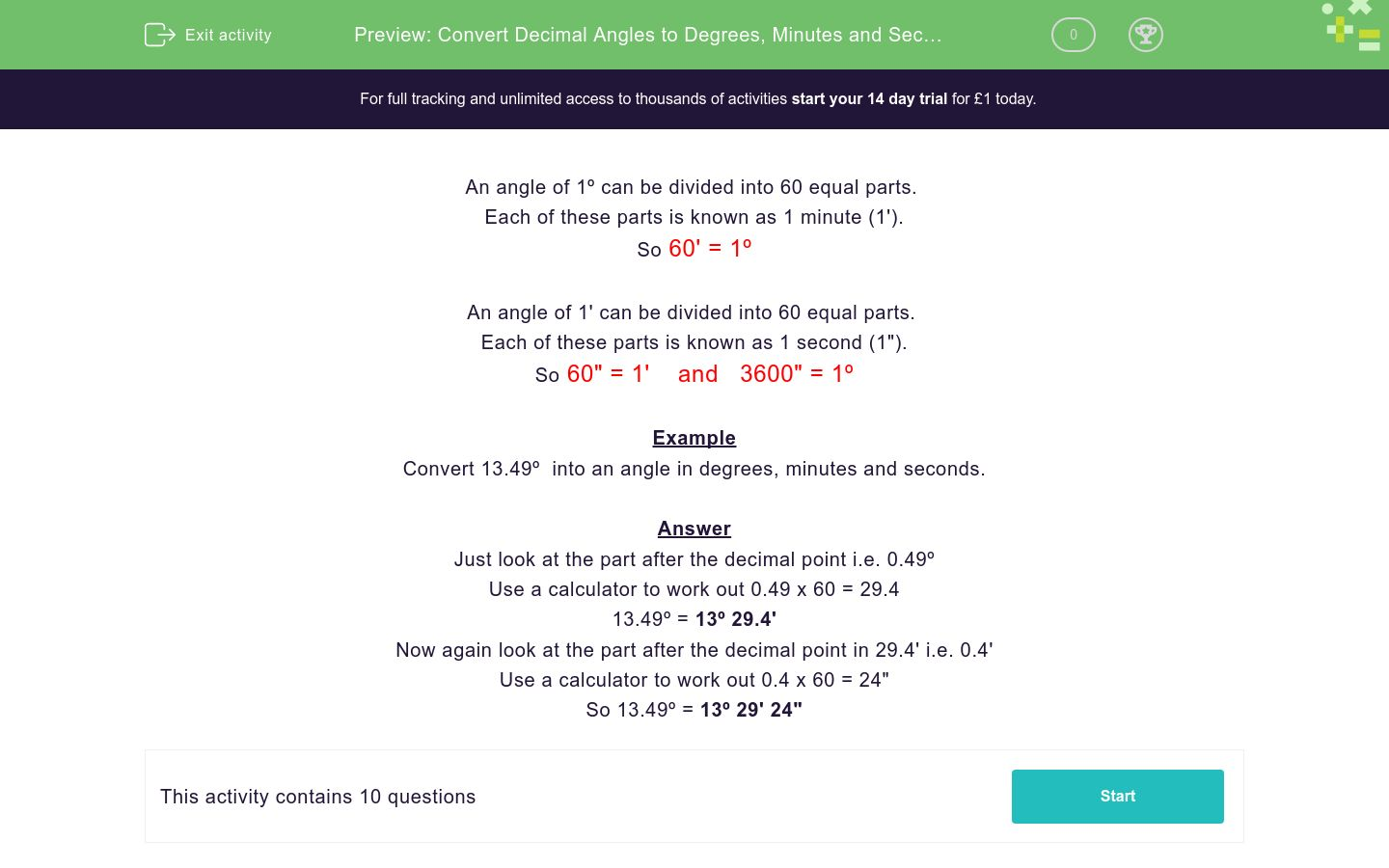# Convert Decimal Angles to Degrees, Minutes and Seconds

In this worksheet, students revise conversion of angles given in decimal format to angles in degrees, minutes and seconds.Key stage:  KS 3

Curriculum topic:   Number

Curriculum subtopic:   Use Standard Units of Measure

Difficulty level:#### Worksheet Overview

An angle of 1º can be divided into 60 equal parts.

Each of these parts is known as 1 minute (1').

So 60' = 1º

An angle of 1' can be divided into 60 equal parts.

Each of these parts is known as 1 second (1").

So 60" = 1'    and   3600" = 1º

Example

Convert 13.49º  into an angle in degrees, minutes and seconds.

Just look at the part after the decimal point i.e. 0.49º

Use a calculator to work out 0.49 x 60 = 29.4

13.49º = 13º 29.4'

Now again look at the part after the decimal point in 29.4' i.e. 0.4'

Use a calculator to work out 0.4 x 60 = 24"

So 13.49º = 13º 29' 24"

### What is EdPlace?

We're your National Curriculum aligned online education content provider helping each child succeed in English, maths and science from year 1 to GCSE. With an EdPlace account you’ll be able to track and measure progress, helping each child achieve their best. We build confidence and attainment by personalising each child’s learning at a level that suits them.

Get started